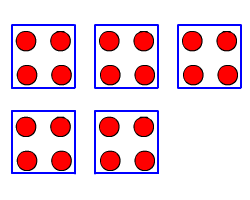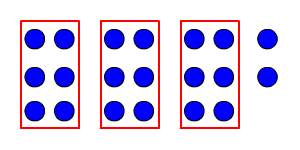# Division: Whole Numbers

Division is a mathematical operation, written using the symbol $÷$ , that can be thought of in two ways: $a÷b$ is the size of each group when $a$ objects are divided into $b$ groups of equal size, OR $a÷b$ is the number of groups when $a$ objects are divided into groups of $b$ objects each.

For example, $20÷4$ can be found by dividing $20$ dots into $4$ groups of equal size.We find that each of the four groups contains $5$ dots, so $20÷4=5$ .

Alternately, we can find $20÷4$ by forming groups of $4$ dots each and then counting how many groups there are:There are $5$ groups.

Division is the inverse operation of multiplication. That is,

$a÷b=c$ if and only if $c×b=a$ .

Here $a$ is called the dividend , $b$ is called the divisor , and $c$ (the result) is called the quotient .

Division by $0$ is undefined; to see why, substitute $b=0$ in the above equations. Since $c×0=0$ no matter what the value of $c$ , so $a$ must also equal $0$ ; and if $a$ and $b$ are both $0$ , $c$ can equal anything!

Division with whole numbers can result in a remainder. For example, if we divide $20÷6$ by dividing $20$ into groups of $6$ , we get $3$ groups with $2$ left over:Sometimes we write $20÷6=3\text{\hspace{0.17em}}\text{\hspace{0.17em}}\text{R}\text{\hspace{0.17em}}\text{\hspace{0.17em}}2$ , where $2$ is the remainder .

Or, we can write a single number answer as a fraction or decimal.

Long Division

To divide a multi-digit number by a one-digit number, we can use long division.

Example 1:

Divide. $496÷8$

Place the dividend inside the division symbol, the divisor on the outside the division symbol.

$8\overline{)496}$

Here, $8$ cannot be contained in $4$ , so consider the next digit also. There are $6$ eights in $49$ , so write $6$ at the tens place of the quotient.

Multiply $6$ by the divisor $8$ and subtract.

$\begin{array}{l}\begin{array}{c}\text{\hspace{0.17em}}\text{\hspace{0.17em}}\text{\hspace{0.17em}}{6}\\ 8\overline{){49}6}\end{array}\hfill \\ \text{\hspace{0.17em}}\text{\hspace{0.17em}}\text{\hspace{0.17em}}\text{\hspace{0.17em}}\text{\hspace{0.17em}}\underset{_}{\text{\hspace{0.17em}}{48}}\hfill \\ \text{\hspace{0.17em}}\text{\hspace{0.17em}}\text{\hspace{0.17em}}\text{\hspace{0.17em}}\text{\hspace{0.17em}}\text{\hspace{0.17em}}\text{\hspace{0.17em}}\text{\hspace{0.17em}}\text{\hspace{0.17em}}{1}\hfill \end{array}$

Now again $8$ cannot be contained in $1$ , so bring down the next digit $6$ .

$\begin{array}{l}\begin{array}{c}\text{\hspace{0.17em}}\text{\hspace{0.17em}}\text{\hspace{0.17em}}6\\ 8\overline{)49{6}}\end{array}\hfill \\ \text{\hspace{0.17em}}\text{\hspace{0.17em}}\text{\hspace{0.17em}}\text{\hspace{0.17em}}\text{\hspace{0.17em}}\underset{_}{\text{\hspace{0.17em}}48}\hfill \\ \text{\hspace{0.17em}}\text{\hspace{0.17em}}\text{\hspace{0.17em}}\text{\hspace{0.17em}}\text{\hspace{0.17em}}\text{\hspace{0.17em}}\text{\hspace{0.17em}}\text{\hspace{0.17em}}{16}\hfill \end{array}$

There $2$ eights in $16$ , so write $2$ at the ones place.

Multiply $2$ by the divisor $8$ and subtract.

$\begin{array}{l}\begin{array}{c}\text{\hspace{0.17em}}\text{\hspace{0.17em}}\text{\hspace{0.17em}}\text{\hspace{0.17em}}\text{\hspace{0.17em}}\text{\hspace{0.17em}}\text{\hspace{0.17em}}6{2}\\ 8\overline{)49{6}}\end{array}\hfill \\ \text{\hspace{0.17em}}\text{\hspace{0.17em}}\text{\hspace{0.17em}}\text{\hspace{0.17em}}\text{\hspace{0.17em}}\underset{_}{\text{\hspace{0.17em}}48}\hfill \\ \text{\hspace{0.17em}}\text{\hspace{0.17em}}\text{\hspace{0.17em}}\text{\hspace{0.17em}}\text{\hspace{0.17em}}\text{\hspace{0.17em}}\text{\hspace{0.17em}}\text{\hspace{0.17em}}{16}\hfill \\ \text{\hspace{0.17em}}\text{\hspace{0.17em}}\text{\hspace{0.17em}}\text{\hspace{0.17em}}\text{\hspace{0.17em}}\text{\hspace{0.17em}}\underset{_}{\text{\hspace{0.17em}}\text{\hspace{0.17em}}{16}}\hfill \\ \text{\hspace{0.17em}}\text{\hspace{0.17em}}\text{\hspace{0.17em}}\text{\hspace{0.17em}}\text{\hspace{0.17em}}\text{\hspace{0.17em}}\text{\hspace{0.17em}}\text{\hspace{0.17em}}\text{\hspace{0.17em}}\text{\hspace{0.17em}}{0}\hfill \end{array}$

To divide a multi-digit number by a multi-digit number, the process is similar.

Example 2:

Divide $1036$ by $32$ . Place the dividend inside the division symbol, the divisor on the outside the division symbol.

$32\overline{)1036}$

Here, $32$ cannot be contained in $1$ , consider the next digit, still $32$ cannot be contained in $10$ . So, consider the next digit also. There are $3$ thirty twos in $103$ , so write $3$ at the tens place of the quotient.

Multiply $3$ by the divisor $32$ and subtract.

$\begin{array}{l}\begin{array}{c}\text{\hspace{0.17em}}\text{\hspace{0.17em}}\text{\hspace{0.17em}}\text{\hspace{0.17em}}\text{\hspace{0.17em}}\text{\hspace{0.17em}}\text{\hspace{0.17em}}\text{\hspace{0.17em}}\text{\hspace{0.17em}}{3}\\ 32\overline{){103}6}\end{array}\hfill \\ \text{\hspace{0.17em}}\text{\hspace{0.17em}}\text{\hspace{0.17em}}\text{\hspace{0.17em}}\text{\hspace{0.17em}}\text{\hspace{0.17em}}\text{\hspace{0.17em}}\underset{_}{\text{\hspace{0.17em}}\text{\hspace{0.17em}}\text{\hspace{0.17em}}\text{\hspace{0.17em}}{96}}\hfill \\ \text{\hspace{0.17em}}\text{\hspace{0.17em}}\text{\hspace{0.17em}}\text{\hspace{0.17em}}\text{\hspace{0.17em}}\text{\hspace{0.17em}}\text{\hspace{0.17em}}\text{\hspace{0.17em}}\text{\hspace{0.17em}}\text{\hspace{0.17em}}\text{\hspace{0.17em}}\text{\hspace{0.17em}}\text{\hspace{0.17em}}{7}\hfill \end{array}$

Now again $32$ cannot be contained in $7$ , so bring down the next digit $6$ .

$\begin{array}{l}\begin{array}{c}\text{\hspace{0.17em}}\text{\hspace{0.17em}}\text{\hspace{0.17em}}\text{\hspace{0.17em}}\text{\hspace{0.17em}}\text{\hspace{0.17em}}\text{\hspace{0.17em}}\text{\hspace{0.17em}}3\\ 32\overline{)1036}\end{array}\hfill \\ \text{\hspace{0.17em}}\text{\hspace{0.17em}}\text{\hspace{0.17em}}\text{\hspace{0.17em}}\text{\hspace{0.17em}}\text{\hspace{0.17em}}\text{\hspace{0.17em}}\underset{_}{\text{\hspace{0.17em}}\text{\hspace{0.17em}}\text{\hspace{0.17em}}\text{\hspace{0.17em}}96}\hfill \\ \text{\hspace{0.17em}}\text{\hspace{0.17em}}\text{\hspace{0.17em}}\text{\hspace{0.17em}}\text{\hspace{0.17em}}\text{\hspace{0.17em}}\text{\hspace{0.17em}}\text{\hspace{0.17em}}\text{\hspace{0.17em}}\text{\hspace{0.17em}}\text{\hspace{0.17em}}\text{\hspace{0.17em}}\text{\hspace{0.17em}}{76}\hfill \end{array}$

There $2$ thirty twos in $76$ , so write $2$ at the ones place.

Multiply $2$ by the divisor $32$ and subtract.

Here the remainder is $12$ .

$\begin{array}{l}\begin{array}{c}\text{\hspace{0.17em}}\text{\hspace{0.17em}}\text{\hspace{0.17em}}\text{\hspace{0.17em}}\text{\hspace{0.17em}}\text{\hspace{0.17em}}\text{\hspace{0.17em}}\text{\hspace{0.17em}}\text{\hspace{0.17em}}\text{\hspace{0.17em}}\text{\hspace{0.17em}}\text{\hspace{0.17em}}3{2}\\ 32\overline{)103{6}}\end{array}\hfill \\ \text{\hspace{0.17em}}\text{\hspace{0.17em}}\text{\hspace{0.17em}}\text{\hspace{0.17em}}\text{\hspace{0.17em}}\text{\hspace{0.17em}}\text{\hspace{0.17em}}\underset{_}{\text{\hspace{0.17em}}\text{\hspace{0.17em}}\text{\hspace{0.17em}}\text{\hspace{0.17em}}96}\hfill \\ \text{\hspace{0.17em}}\text{\hspace{0.17em}}\text{\hspace{0.17em}}\text{\hspace{0.17em}}\text{\hspace{0.17em}}\text{\hspace{0.17em}}\text{\hspace{0.17em}}\text{\hspace{0.17em}}\text{\hspace{0.17em}}\text{\hspace{0.17em}}\text{\hspace{0.17em}}\text{\hspace{0.17em}}\text{\hspace{0.17em}}{76}\hfill \\ \text{\hspace{0.17em}}\text{\hspace{0.17em}}\text{\hspace{0.17em}}\text{\hspace{0.17em}}\text{\hspace{0.17em}}\text{\hspace{0.17em}}\text{\hspace{0.17em}}\text{\hspace{0.17em}}\text{\hspace{0.17em}}\text{\hspace{0.17em}}\text{\hspace{0.17em}}\underset{_}{\text{\hspace{0.17em}}\text{\hspace{0.17em}}{64}}\hfill \\ \text{\hspace{0.17em}}\text{\hspace{0.17em}}\text{\hspace{0.17em}}\text{\hspace{0.17em}}\text{\hspace{0.17em}}\text{\hspace{0.17em}}\text{\hspace{0.17em}}\text{\hspace{0.17em}}\text{\hspace{0.17em}}\text{\hspace{0.17em}}\text{\hspace{0.17em}}\text{\hspace{0.17em}}\text{\hspace{0.17em}}{12}\hfill \end{array}$

Unlike addition and multiplication, for real numbers , the division operation is not commutative . That is, order matters: $40÷8=5$ , but $8÷40=\frac{1}{5}$ (a fractional value).

Similarly, division is not associative ; that is, grouping matters. For instance,

$\left(80÷10\right)÷2=8÷2=4,\text{\hspace{0.17em}}\text{\hspace{0.17em}}\text{but}$

$80÷\left(10÷2\right)=80÷5=16$ .